# Making heat maps with blender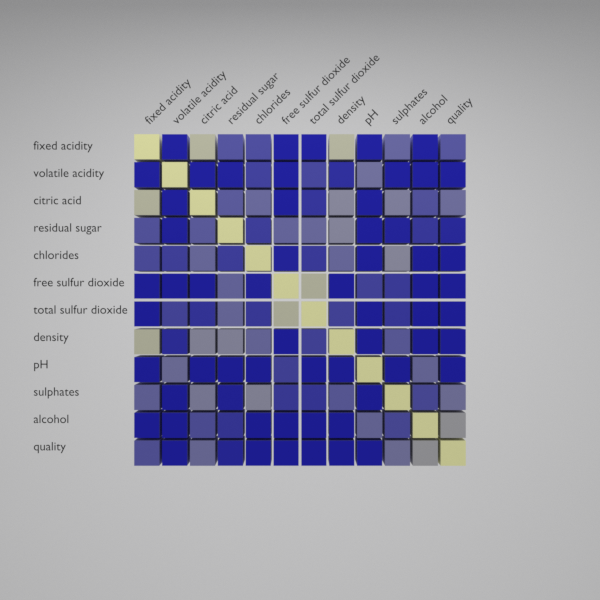Image by author

Displaying a matrix with a heat map is one type of data visualization that allows us to understand pairwise relationships among features. A heat map is a data visualization technique where the data is encoded by the change in color. One of the most common uses of the heat map is to represent the correlation between features. With pandas, the calculation of the correlation between features is really simple. And also saving that data to a CSV file is a matter of a single line of code.

Displaying the correlation with a heat map results in a square matrix, with a size equal to the number of features being analyzed.

Depending on the color map used the difference between values can be easily spotted or not, however, the main diagonal of the matrix gave us the top reference value, as the corresponding feature is being compared to itself. For correlation, the diagonal value will be one and that will be the highest value in the visualization. If the heat map displays a distance measure then the diagonal value will be zero as the distance between two equal points is zero. The previous description will be valid if both rows and columns represent the same features otherwise the main diagonal will display a different color.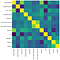Image by author

With some of the basics of a color map already covered and a simple way to create that plot in python, we can start to adapt the classic visualization to blender. The heat map body can be seen as a two-dimensional grid and each element of the grid is a square in the heat map. From that grid, we need to obtain the mid-point coordinates. That grid is composed of N+1 divisions on the x-axis and y-axis. For each pair of segments in the grid, the central point contains the location of the geometry that needs to be added. Then each central point needs to be generated from top to bottom and from left to right, just in the same order as the data in the CSV file.

By doing that, the amount of post-processing of the data is minimized as the data can be added without any reindexing. Then to add the geometry a simple option will be added to control the size of the geometry, that argument could be a single value or an array with the same size as the number of objects. By doing so we can add another layer of encoding to the visualization by manipulating both color and shape.

Know that the geometry can be added in the proper location a material needs to be added to modify color in the object. The material will change how the light is absorbed or bounces over the object, however, the encoded information will be in the color used for that object.

A quick render of the different objects in the scene results in the following.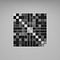Image by author

Both geometry and materials can be added in the correct location and without any warning, however, the labels of the data are missing. To fix that we create a simple function to add text into the scene, once the text element is in the scene the default text is erased and the label is added. Then we apply some simple rotations so the text is aligned to the camera.

With all in place, we can render the final visualization and obtain the following. With the same scale on each of the geometries, the interpretation of that visualization falls directly into the color map used, however when wrong or difficult to understand colormap is used the size encoding can help us to get a better understanding of the data.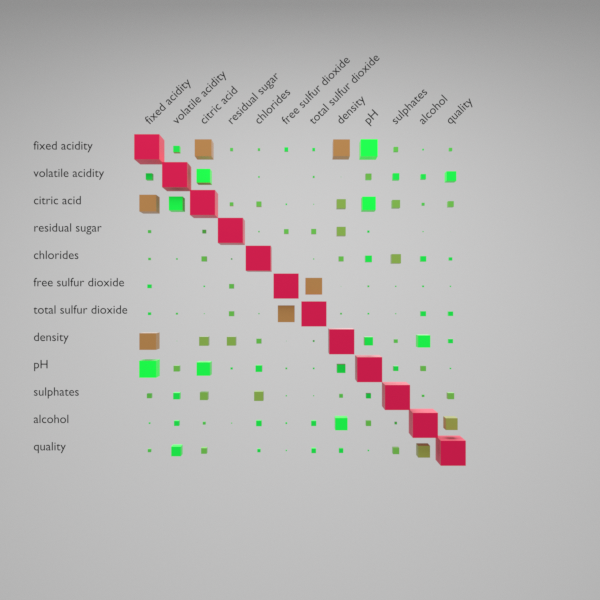Image by author

Besides correlation between features, the matrix visualization can be used to represent the similarity between different features. We can segment the data by its unique quality values and measure its similarity to the mean value.

As sometimes the similarity or dissimilarity values between two samples could be small, then the resulting color value will not be helpful to look for patterns, thus a min-max normalization will be helpful to make comparisons easier.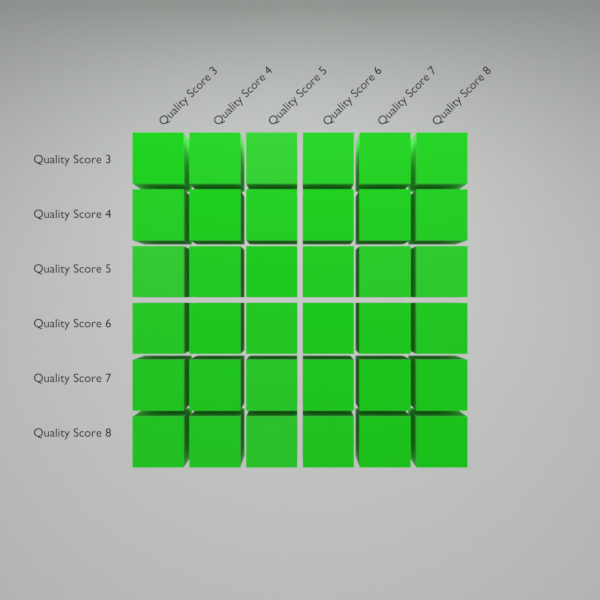Image by author

Know you have a small example of how to recreate a commonly used data visualization in blender, as well as some design considerations. Also, the importance of the colour map to make assessments of the data and how helpful are some other forms of encoding. The complete code for this post can be found on my Github by clicking here. And the data can be downloaded from the UCI Machine Learning Repository by clicking here. See you in the next one.

Writing about math, natural sciences and academia.